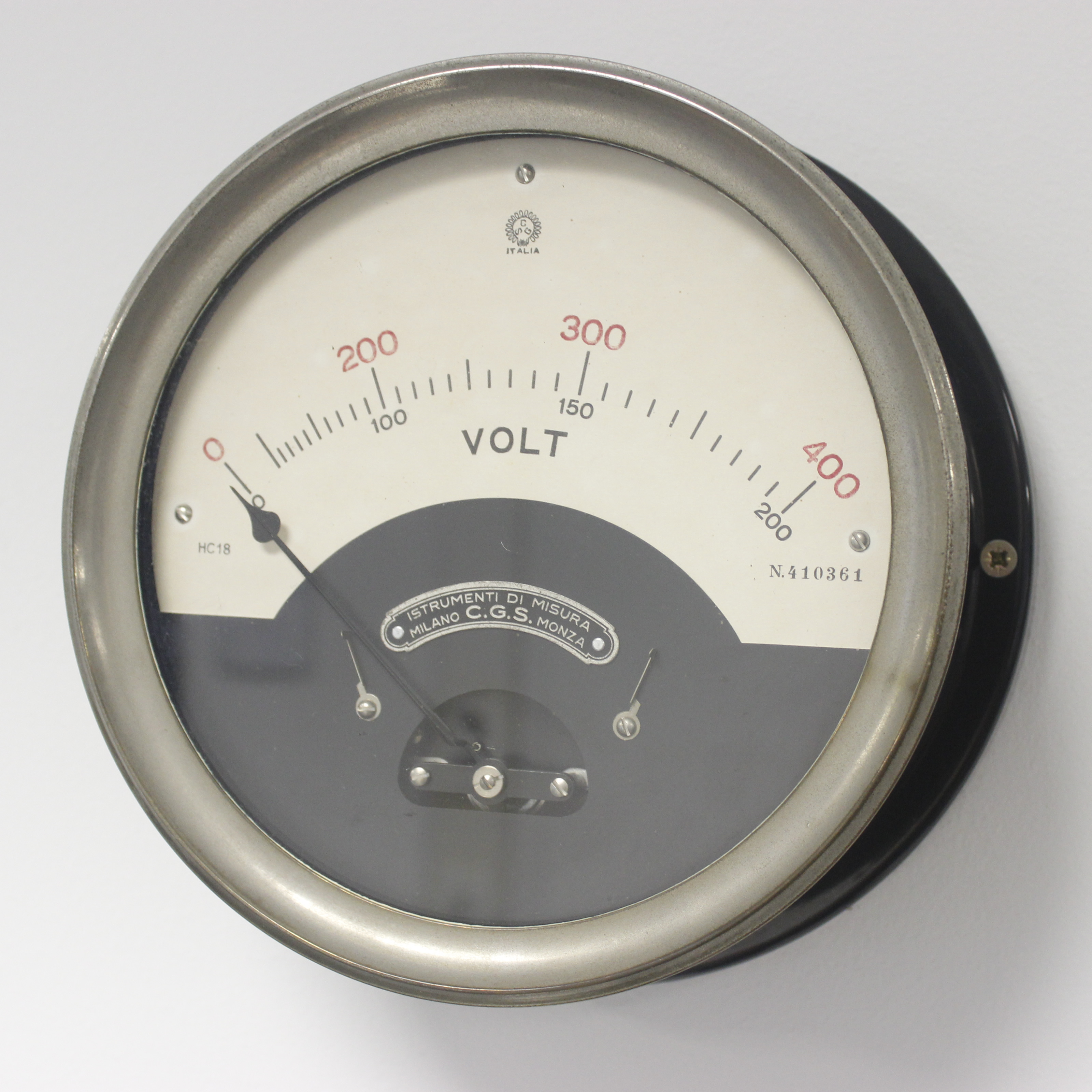# VOLTMETERA voltmeter, also known as a voltage meter, is an instrument used to measure the potential difference, or voltage, between two points in an electrical or electronic circuit. Some voltmeters are intended for use in direct current (DC) circuits; others are designed for alternating current (AC) circuits. Specialized voltmeters can measure radio frequency (RF) voltage.
Measurements are made on a scale normally graduated in millivolts (0.001 volts), volts or kilovolts (1,000 volts).

## INSIGHTS

An analog voltmeter consists of a very sensitive galvanometer (current meter) connected in series to a resistance of adequate value. The overall resistance must be high, otherwise the instrument will draw a significant current and disrupt the operation of the circuit under test. The sensitivity of the galvanometer and the value of the series resistance determine the range of voltages that the instrument can measure.
The electronic voltmeter, which has largely replaced the vacuum tube voltmeter, uses active circuits powered by an external source ( batteries or mains); the current required to activate the galvanometer movement is not taken from the circuit to be measured but supplied by the active circuits; therefore, this type of instrument does not disturb the circuit under test.
Today mainly digital voltmeters are used, which show the voltage directly in the form of numbers. Some of these meters can determine voltage values ​​across multiple significant digits. Practical laboratory voltmeters have a maximum range between 1000 and 3000 volts. The more sophisticated tools also provide output that can be transmitted remotely, can activate printers, and can be connected to computers. Digital voltmeters also generally have higher accuracy than analog instruments.
An instrument that also measures resistance (ohms) and current (ampere) values ​​is called a multimeter.